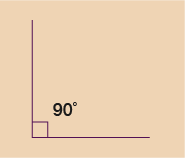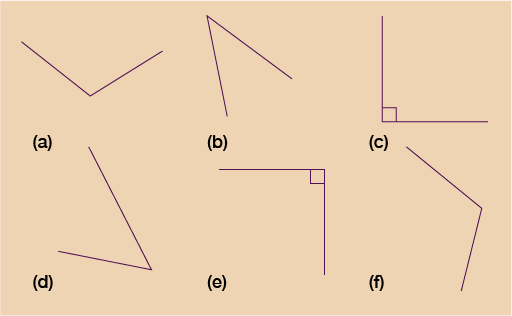Everyday maths 1 (Wales)

Start this free course now. Just create an account and sign in. Enrol and complete the course for a free statement of participation or digital badge if available.

Free course

# 1.2 Angles

An angle is formed where two straight lines (or sides) meet. Angles are measured in degrees, which is shown by using the symbol ° after the number of degrees. So for example, 45° means an angle of 45 degrees.

Note: Do not confuse these with degrees Celsius, centigrade or Fahrenheit, which are used to measure temperature.

There are 360° in a circle. There are 180° in a half-turn – that is, from north to south on a compass, or from 9 to 3 on a clock.

An angle of 90° is a quarter-turn – from north to east on a compass, or from 12 to 3 on a clock. These angles are also known as right angles. Right angles are shown like this:Figure 3 A right angle

Right angles are very common in everyday life. Look around you and see how many you can spot.

Here are a few examples of where you might have noticed a right angle:

• the corners of your screen (a corner is where two lines meet)
• corners of windows
• the corners of a book page
• where the walls meet the floor
• where the table legs meet the top.

Angles of less than 90° are called acute angles. Angles of more than 90° are called obtuse angles.

## Activity 2: Angles

Which angles in Figure 4 are right angles, acute angles or obtuse angles?Figure 4 Angles

Angles (a) and (f) are obtuse angles (greater than 90°).

Angles (b) and (d) are acute angles (less than 90°).

Angles (c) and (e) are right angles (exactly 90°).

FSM_1_CYMRU#### Live in Wales? Free your ambition with a paid part-time course with the OU in Wales.

With grants of up to £4,500* to help with living costs, and tuition fee loans to cover course fees,
now's the time to take that next step.

#### Find out more

*Eligibility rules apply for financial support.